User:IssaRice/Computability and logic/Eliezer Yudkowsky's Löb's theorem puzzle

This page gives spoilers for the puzzle!

This pages states the puzzle and gives the solution.

Notation

Precedence:

•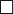$\Box$ has high precedence, so e.g.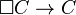$\Box C \to C$ means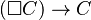$(\Box C) \to C$, and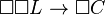$\Box \Box L \to \Box C$ means$(\Box \Box L) \to (\Box C)$.
•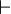$\vdash$ is a metalogical notion and has low precedence, so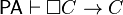$\mathsf{PA} \vdash \Box C \to C$ means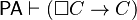$\mathsf{PA} \vdash (\Box C \to C)$.

Translating the puzzle using logic notation

Löb's theorem shows that if$\mathsf{PA} \vdash \Box C \to C$, then$\mathsf{PA} \vdash C$.

The deduction theorem says that if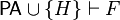$\mathsf{PA} \cup \{H\} \vdash F$, then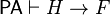$\mathsf{PA} \vdash H \to F$.

Applying the deduction theorem to Löb's theorem gives us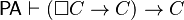$\mathsf{PA} \vdash (\Box C \to C) \to C$.

When translating to logic notation, it becomes obvious that the application of the deduction theorem is illegitimate, because we don't actually have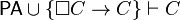$\mathsf{PA} \cup \{\Box C \to C\} \vdash C$. This is the initial answer that Larry D'Anna gives in comments.

But now, suppose we define$\mathsf{PA}' := \mathsf{PA} \cup \{\Box C \to C\}$, and walk through the proof of Löb's theorem for this new theory$\mathsf{PA}'$. Then we would obtain the following implication: if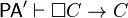$\mathsf{PA}' \vdash \Box C \to C$, then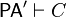$\mathsf{PA}' \vdash C$. But clearly,$\mathsf{PA}' \vdash \Box C \to C$ since$\Box C \to C$ is one of the axioms of$\mathsf{PA}'$. Therefore by modus ponens, we have$\mathsf{PA}' \vdash C$, i.e.$\mathsf{PA}\cup\{\Box C \to C\} \vdash C$. Now we can apply the deduction theorem to obtain$\mathsf{PA} \vdash (\Box C \to C) \to C$. This means that our "Löb's theorem" for$\mathsf{PA}'$ must be incorrect (note: the proof is correct for$\mathsf{PA}$, which is why Löb's theorem is a theorem; it's just incorrect for$\mathsf{PA}'$), and somewhere in the ten-step proof is an error.

Translating the Löb's theorem back to logic

Since the solution to the puzzle refers back to the proof of Löb's theorem, we first translate the proof from the cartoon version back to logic:

1.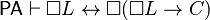$\mathsf{PA} \vdash \Box L \leftrightarrow \Box(\Box L \to C)$ by construction of$L$
2.$\mathsf{PA} \vdash \Box C \to C$ by hypothesis
3.$\mathsf{PA} \vdash \Box(\Box L \to C) \to (\Box \Box L \to \Box C)$
4.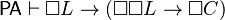$\mathsf{PA} \vdash \Box L \to (\Box \Box L \to \Box C)$
5.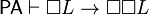$\mathsf{PA} \vdash \Box L \to \Box \Box L$
6.$\mathsf{PA} \vdash \Box L \to \Box C$
7.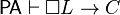$\mathsf{PA} \vdash \Box L \to C$
8.$\mathsf{PA} \vdash \Box(\Box L \to C)$
9.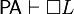$\mathsf{PA} \vdash \Box L$
10.$\mathsf{PA} \vdash C$

Repeating the proof of Löb's theorem for modified theory

We now repeat the proof of Löb's theorem for$\mathsf{PA}' := \mathsf{PA} \cup \{\Box C \to C\}$ to see where the error is.

1.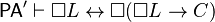$\mathsf{PA}' \vdash \Box L \leftrightarrow \Box(\Box L \to C)$ by construction of$L$
2.$\mathsf{PA}' \vdash \Box C \to C$ because$\Box C \to C$ is one of the axioms of$\mathsf{PA}'$
3.$\mathsf{PA}' \vdash \Box(\Box L \to C) \to (\Box \Box L \to \Box C)$
4.$\mathsf{PA}' \vdash \Box L \to (\Box \Box L \to \Box C)$
5.$\mathsf{PA}' \vdash \Box L \to \Box \Box L$
6.$\mathsf{PA}' \vdash \Box L \to \Box C$
7.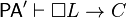$\mathsf{PA}' \vdash \Box L \to C$

So far, everything is fine. But can we assert$\mathsf{PA}' \vdash \Box(\Box L \to C)$? For$\mathsf{PA}$, we had the following:

A1: For each sentence$X$, if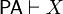$\mathsf{PA}\vdash X$ then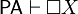$\mathsf{PA} \vdash \Box X$

We need the following:

A1′: For each sentence$X$, if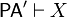$\mathsf{PA}'\vdash X$ then$\mathsf{PA}' \vdash \Box X$

Since$\mathsf{PA}'$ has more axioms than$\mathsf{PA}$, we know that$\mathsf{PA}'$ can prove everything that$\mathsf{PA}$ can. Thus, compared to A1, both the antecedent and consequent of A1′ are stronger, so A1′ does not necessarily follow from A1.

Suppose we take$X$ in A1′ to be$\Box C \to C$. Then we obtain

If$\mathsf{PA}'\vdash \Box C \to C$ then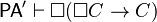$\mathsf{PA}' \vdash \Box (\Box C \to C)$

Other ways we might try to get step 8 to work:

•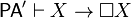$\mathsf{PA}' \vdash X \to \Box X$
• if$X$, then$\mathsf{PA} \vdash X$ -- i think this is the one about which Larry says "ARGH WHAT ARE YOU DOING"

But can we prove that we can never get step 8 for$\mathsf{PA}'$? Well, this is exactly what the puzzle statement does -- it assumes the proof goes through, and derives an absurdity! (? actually, i'm not sure this is actually a contradiction. If we assume PA is consistent, then it seems to be a contradiction. I think this is the point nate soares and alex flint are trying to make in the comments. but we can re-word the puzzle to say one should find the first error.)

Other questions to answer

• does$\Box$ in$\mathsf{PA}'$ still mean provability about$\mathsf{PA}$, or about itself?
• are we removing a layer or adding one?
• can't$\mathsf{PA}'$ prove everything$\mathsf{PA}$ can? yes, but this doesn't mean we can substitute implications automatically because the consequent also gets stronger